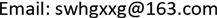﻿ 二阶半正微分方程三点边值问题的正解 Positive Solution for Second-Order Singular Semipositone Differential Equations Three-Point Boundary Value Problems

Pure Mathematics
Vol.08 No.05(2018), Article ID:26550,8 pages
10.12677/PM.2018.85062

Positive Solution for Second-Order Singular Semipositone Differential Equations Three-Point Boundary Value Problems

Jifa Chen

Jining Technician College, Jining ShandongReceived: Aug. 2nd, 2018; accepted: Aug. 16th, 2018; published: Aug. 23rd, 2018ABSTRACT

Using the Krasnoselskii’s fixed point theorem on compression and expansion of cone, this paper investigates a class of second-order singular semipositone differential equations with three-point boundary value problems; a sufficient and the existent range of positive solutions are given.

Keywords:Three-Point Boundary Value Problems, Positive Solution, Fixed Point, SemipositoneCopyright © 2018 by author and Hans Publishers Inc.

This work is licensed under the Creative Commons Attribution International License (CC BY).

http://creativecommons.org/licenses/by/4.0/1. 引言

$\left\{\begin{array}{l}-{u}^{″}+{k}^{2}u=f\left(t,u\right),0 (1)

$I=\left[0,1\right],J=\left[0,1\right],{R}^{+}=\left(0,+\infty \right]$ ，一个函数 $u\left(t\right)\in P{C}^{1}\left(I,R\right)\cap {C}^{2}\left({J}^{1},R\right)$ 称为方程(1)的解，是指满足方程(1)的各项条件，若 $u\left(t\right)$ 在J上恒正，则称为正解。

2. 预备知识

$K\left(t,s\right)$$-{u}^{″}+{k}^{2}u=f\left(t,u\right),u\left(0\right)=\beta u\left(\eta \right),u\left(1\right)=0$ 格林函数， $G\left(t,s\right)-{u}^{″}+{k}^{2}u=f\left(t,u\right)$$u\left(0\right)=\beta u\left(\eta \right),u\left(1\right)=0$ 的格林函数，则 $\mathrm{sinh}\left(k\right)\ne \mathrm{sinh}\left(k\left(1-\eta \right)\right)$ 时，

$K\left(t,s\right)=G\left(t,s\right)+\frac{\mathrm{sinh}\left(k\left(1-t\right)\right)}{\mathrm{sinh}\left(k\right)-\beta \mathrm{sinh}\left(k\left(1-\eta \right)\right)}\beta G\left(\eta ,s\right)$(2)

$G\left(t,s\right)=\left\{\begin{array}{l}\frac{\mathrm{sinh}\left(ks\right)\mathrm{sinh}\left(k\left(1-t\right)\right)}{\mathrm{sinh}\left(k\right)k},0 (3)

i) $\frac{k}{\mathrm{sinh}\left(k\right)}t\left(1-t\right)\le G\left(t,t\right)\le \frac{\mathrm{sinh}\left(k\right)}{k}t\left(1-t\right)\le \frac{\mathrm{sinh}\left(k\right)}{k}$

ii) $\frac{k}{\mathrm{sinh}\left(k\right)}G\left(t,t\right)\le G\left(s,s\right)\le G\left(t,s\right)\le G\left(t,t\right)$

iii)当 $\mathrm{sinh}\left(k\right)-\beta \mathrm{sinh}\left(k\left(1-\eta \right)\right)>0$$mt\left(1-t\right)s\left(1-s\right)\le K\left(t,s\right)\le Ms\left(1-s\right)$ 。这里 $M=\left(\frac{\mathrm{sinh}\left(k\right)\left(1+\beta \right)-\beta \mathrm{sinh}\left(k\left(1-\eta \right)\right)}{\mathrm{sinh}\left(k\right)-\beta \mathrm{sinh}\left(k\left(1-\eta \right)\right)}\right)\frac{\mathrm{sinh}\left(k\right)}{k},m=\frac{{k}^{3}}{\mathrm{sinh}{\left(k\right)}^{3}}$

iv) 当 $\mathrm{sinh}\left(k\right)-\beta \mathrm{sinh}\left(k\left(1-\eta \right)\right)>0$ 时， ${M}_{1}\left(1-t\right)G\left(s,s\right)\le K\left(t,s\right)\le {M}_{2}\left(1-t\right)$ ，其中 ${M}_{1}=\frac{\beta k}{\mathrm{sinh}\left(k\right)-\beta \mathrm{sinh}\left(k\left(1-\eta \right)\right)}\frac{k}{\mathrm{sinh}\left(k\right)}G\left(\eta ,\eta \right)$${M}_{2}=\frac{\mathrm{sinh}\left(k\right)}{k}+\frac{\beta \mathrm{sinh}\left(k\right)}{\mathrm{sinh}\left(k\right)-\beta \mathrm{sinh}\left(k\left(1-\eta \right)\right)}G\left(\eta ,\eta \right)$

$kt\le \mathrm{sinh}\left(kt\right)\le \mathrm{sinh}\left(k\right)t,t\in \left[0,1\right]$(4)

$K\left(t,s\right)\ge G\left(t,s\right)\ge \frac{\mathrm{sinh}\left(k\right)}{k}G\left(s,s\right)\ge \frac{{k}^{3}}{\mathrm{sinh}{\left(k\right)}^{3}}t\left(1-t\right)s\left(1-s\right)$

$\begin{array}{c}K\left(t,s\right)\le G\left(s,s\right)+\frac{\mathrm{sinh}\left(k\left(1-t\right)\right)}{\mathrm{sinh}\left(k\right)-\beta \mathrm{sinh}\left(k\left(1-\eta \right)\right)}\beta G\left(s,s\right)\\ =1+\left(\frac{\mathrm{sinh}\left(k\left(1-t\right)\right)}{\mathrm{sinh}\left(k\right)-\beta \mathrm{sinh}\left(k\left(1-\eta \right)\right)}\beta \right)G\left(s,s\right)\\ \le 1+\left(\frac{\mathrm{sinh}\left(k\right)\beta }{\mathrm{sinh}\left(k\right)-\beta \mathrm{sinh}\left(k\left(1-\eta \right)\right)}\right)\frac{\mathrm{sinh}\left(k\right)}{k}s\left(1-s\right)\\ =Ms\left(1-s\right)\end{array}$

$K\left(t,s\right)\ge \frac{\beta k}{\mathrm{sinh}\left(k\right)-\beta \mathrm{sinh}\left(k\left(1-\eta \right)\right)}\cdot \frac{k}{\mathrm{sinh}\left(k\right)}G\left(\eta ,\eta \right)G\left(s,s\right)\left(1-t\right)={M}_{1}=\left(1-t\right)G\left(s,s\right)$ $K\left(t,s\right)\le \frac{k}{\mathrm{sinh}\left(k\right)}t\left(1-t\right)+\frac{\beta \mathrm{sinh}\left(k\right)}{\mathrm{sinh}\left(k\right)-\beta \mathrm{sinh}\left(k\left(1-\eta \right)\right)}G\left(\eta ,\eta \right)\left(1-t\right)\le {M}_{2}\left(1-t\right)$

${m}_{2}t\left(1-t\right)s\left(1-s\right)\le K\left(t,s\right)\le {M}_{2}\left(1-t\right)$ (5)

$‖Au‖\le ‖u‖,\forall u\in P\cap \theta {\Omega }_{1}‖Au‖\ge ‖u‖,\forall u\in P\cap \theta {\Omega }_{2}$

$E=C\left[0,1\right],‖u‖=\underset{t\in \left[0,1\right]}{\mathrm{max}}|u\left(t\right)|$$P=\left\{u|u\left(t\right)\ge 0,u\in E\right\}$$Q=\left\{u|u\in P,u\left(t\right)\ge \epsilon ‖u‖e\left(t\right),t\in I\right\}$

3. 主要结果及证明

(H1) 存在函数 $p,q\in C\left(J,{R}^{+}\right),h\in C\left({R}^{+},{R}^{+}\right),g\left(t,u\right)\in C\left(J×{R}^{+},{R}^{+}\right)$ 使得

$g\left(t,u\right)-q\left(t\right)\le f\left(t,u\right)\le p\left(t\right)h\left(u\right),\forall t\in J,u\in {R}^{+}$

(H2) 存在闭区间 $\left[a,b\right]\subset I$ ,使得 $\underset{u\to +\infty }{\mathrm{lim}}\frac{g\left(t,u\right)}{u}=+\infty$$\left[a,b\right]$ 上一致成立。

(H3) 存在 $r>0$ ，使得下面两个式子成立：

$0<\underset{0}{\overset{1}{\int }}q\left(s\right)\text{d}s<\frac{\epsilon r}{{M}_{2}}$ (6)

$0<\underset{0}{\overset{1}{\int }}p\left(s\right)+q\left(s\right)\text{d}s<\frac{r}{M\underset{0\le \tau \le t}{\mathrm{max}}\left\{h\left(\tau \right),1\right\}}$(7)

$e\left(t\right)\ge e\left[a,b\right]>0,t\in \left[a,b\right]$(8)

${x}_{0}\left(t\right)=\underset{0}{\overset{1}{\int }}K\left(t,s\right)q\left(s\right)\text{d}s,t\in I$(9)

$0\le {x}_{0}\left(t\right)\le \underset{0}{\overset{1}{\int }}Ms\left(1-s\right)q\left(s\right)\text{d}s\le \underset{0}{\overset{1}{\int }}Mq\left(s\right)\text{d}s,t\in I$(10)

$\left\{\begin{array}{l}-{{x}^{″}}_{0}+{k}^{2}{x}_{0}=q\left(t\right),0 (11)

${M}_{3}e\left(t\right)\le \omega \left(t\right)\le {M}_{4}e\left(t\right),t\in I$(12)

${\left[k\left(t\right)\right]}_{+}=\left\{\begin{array}{l}kt,k\left(t\right)\ge 0,\\ 0,k\left(t\right)\le 0.\end{array}$

$Au\left(t\right)=\underset{0}{\overset{1}{\int }}K\left(t,s\right)f\left(s,{\left[u\left(s\right)-{x}_{0}\left(s\right)\right]}_{+}\right)+q\left(s\right)\text{d}s,\forall u\left(t\right)\in P$ (13)

$\begin{array}{l}f\left(s,{\left[u\left(s\right)-{x}_{0}\left(s\right)\right]}_{+}\right)+q\left(s\right)p\left(s\right)h\left({\left[u\left(s\right)-{x}_{0}\left(s\right)\right]}_{+}\right)+q\left(s\right)\\ \le \underset{0\le \tau \le ‖u‖}{\mathrm{max}}\left\{h\left(\tau \right),1\right\}\left(p\left(s\right)+q\left(s\right)\right),\forall s\in J\end{array}$ (14)

$\begin{array}{l}\underset{0}{\overset{1}{\int }}K\left(t,s\right)\left(f\left(s,{\left[u\left(s\right)-{x}_{0}\left(s\right)\right]}_{+}+q\left(s\right)\right)\right)\text{d}s\\ \le {M}_{2}e\left(t\right)\underset{0\le \tau \le ‖u‖}{\mathrm{max}}\left\{h\left(\tau \right),1\right\}\underset{0}{\overset{1}{\int }}\left(p\left(s\right)+q\left(s\right)\right)\text{d}s\\ \le {M}_{2}\underset{0\le \tau \le ‖u‖}{\mathrm{max}}\left\{h\left(\tau \right),1\right\}\underset{0}{\overset{1}{\int }}\left(p\left(s\right)+q\left(s\right)\right)\text{d}s\le +\infty ,t\in I\end{array}$ (15)

i) A为映Q到Q的全连续算子

$f\left(s,{\left[u\left(s\right)-{x}_{0}\left(s\right)\right]}_{+}\right)+q\left(s\right)\ge g\left(s,{\left[u\left(s\right)-{x}_{0}\left(s\right)\right]}_{+}\right)\ge 0,s\in J$

$Ay\left(t\right)\le M\underset{0}{\overset{1}{\int }}s\left(1-s\right)\left(f\left(s,{\left[u\left(s\right)-{x}_{0}\left(s\right)\right]}_{+}\right)+q\left(s\right)\right)\text{d}s$

$‖y‖\le M\underset{0}{\overset{1}{\int }}s\left(1-s\right)\left(f\left(s,{\left[u\left(s\right)-{x}_{0}\left(s\right)\right]}_{+}\right)+q\left(s\right)\right)\text{d}s$

$y\left(t\right)\ge \underset{0}{\overset{1}{\int }}{m}_{2}e\left(t\right)s\left(1-s\right)\left(f\left(s,{\left[u\left(s\right)-{x}_{0}\left(s\right)\right]}_{+}\right)+q\left(s\right)\right)\text{d}s\ge \frac{{m}_{2}}{M}‖y‖e\left(t\right)=\epsilon ‖y‖e\left(t\right)$

$D\subset Q$ 是有界集，于是存在常数 ${L}_{1}>0$ ，使得 $\forall u\in D,‖u‖\le {L}_{1}$ ，且

${\left[u\left(s\right)-{x}_{0}\left(s\right)\right]}_{+}\le u\left(s\right)\le {L}_{1}$

$‖Au‖\le M\underset{0\le \tau \le ‖u‖}{\mathrm{max}}\left\{h\left(\tau \right),1\right\}\underset{0}{\overset{1}{\int }}s\left(1-s\right)\left(p\left(s\right)+q\left(s\right)\right)\text{d}s\le M\underset{0\le \tau \le ‖u‖}{\mathrm{max}}\left\{h\left(\tau \right),1\right\}\underset{0}{\overset{1}{\int }}\left(p\left(s\right)+q\left(s\right)\right)\text{d}s<+\infty$ 即A(D)一致有界。

$|K\left({t}_{1},s\right)-K\left({t}_{2},s\right)|<\epsilon {\left(\underset{0\le \tau \le ‖u‖}{\mathrm{max}}\left\{h\left(\tau \right),1\right\}\underset{0}{\overset{1}{\int }}\left(p\left(s\right)+q\left(s\right)\right)\text{d}s\right)}^{-1}$

$\begin{array}{l}|Au\left({t}_{1}\right)-Au\left({t}_{2}\right)|\\ =\underset{0}{\overset{1}{\int }}|K\left({t}_{1},s\right)-K\left({t}_{2},s\right)|f\left(s,{\left[u\left(s\right)-{x}_{0}\left(s\right)\right]}_{+}\right)+q\left(s\right)\text{d}s\\ \le \underset{0\le \tau \le ‖u‖}{\mathrm{max}}\left\{h\left(\tau \right),1\right\}\underset{0}{\overset{1}{\int }}|K\left({t}_{1},s\right)-K\left({t}_{2},s\right)|\left(p\left(s\right)+q\left(s\right)\right)\text{d}s<\epsilon \end{array}$

${u}_{n},{u}_{0}\in Q,{u}_{n}\to {u}_{0}\left(n\to +\infty \right)$ ，则 $\left\{{u}_{n}\right\}$ 有界。令 ${L}_{2}=\mathrm{sup}\left\{‖{u}_{n}‖,n=0,1,2,\cdots \right\}$ ，则

$\begin{array}{l}f\left(s,{\left[{u}_{n}\left(s\right)-{x}_{0}\left(s\right)\right]}_{+}\right)+q\left(s\right)\\ \le \underset{0\le \tau \le ‖u‖}{\mathrm{max}}\left\{h\left(\tau \right),1\right\}\left(p\left(s\right)+q\left(s\right)\right),n=0,1,2,\cdots \end{array}$ (16)

$\begin{array}{l}|A{u}_{n}\left(t\right)-A{u}_{0}\left(t\right)|\\ \le M\underset{0}{\overset{1}{\int }}s\left(1-s\right)|\left(f\left(s,{\left[{u}_{n}\left(t\right)-{x}_{0}\left(t\right)\right]}_{+}\right)-f\left(s,{\left[{u}_{0}\left(t\right)-{x}_{0}\left(t\right)\right]}_{+}\right)\right)|\text{d}s\\ \le M\underset{0}{\overset{1}{\int }}|\left(f\left(s,{\left[{u}_{n}\left(t\right)-{x}_{0}\left(t\right)\right]}_{+}\right)-f\left(s,{\left[{u}_{0}\left(t\right)-{x}_{0}\left(t\right)\right]}_{+}\right)\right)|\text{d}s,n=0,1,2,\cdots \end{array}$ (17)

ii) 算子A在Q中有不动点

$\forall x\in \partial {\Omega }_{r}\cap Q$ ，由(14)式得到

$\begin{array}{l}Ax\left(t\right)=\underset{0}{\overset{1}{\int }}K\left(t,s\right)f\left(s,{\left[x\left(s\right)-{x}_{0}\left(s\right)\right]}_{+}\right)+q\left(s\right)\text{d}s\\ \le \underset{0\le \tau \le ‖u‖}{M\mathrm{max}}\left\{h\left(\tau \right),1\right\}\underset{0}{\overset{1}{\int }}\left(p\left(s\right)+q\left(s\right)\right)\text{d}s\end{array}$

$‖Ax‖\le r=‖x‖,\forall x\in \partial {\Omega }_{r}\cap Q$(18)

$\underset{0}{\overset{1}{\int }}q\left(s\right)\text{d}s<\frac{{m}_{0}}{{m}_{0}+1}\frac{\epsilon r}{{M}_{2}}$ (19)

$L>\left({m}_{0}+1\right){\left(\epsilon e\left[a,b\right]\underset{t\in I}{\mathrm{max}}\underset{a}{\overset{b}{\int }}K\left(t,s\right)\text{d}s\right)}^{-1}$

$g\left(t,u\right)\ge Lu,\forall t\in \left[a,b\right],u\ge {R}_{1}$ (20)

$R>\mathrm{max}\left\{r,\frac{\left({m}_{0}+1\right){R}_{1}}{\epsilon e\left[a,b\right]}\right\}$ (21)

$‖Ay‖\ge ‖y‖,\forall y\in \partial {\Omega }_{R}\cap Q$ (22)

$\forall y\in \partial {\Omega }_{R}\cap Q$ ，有 $y\left(t\right)\ge \epsilon ‖y‖e\left(t\right)$ 。于是由(8) (19)式得到

$\begin{array}{c}{x}_{0}\left(t\right)\le {M}_{2}e\left(t\right)\underset{0}{\overset{1}{\int }}q\left(s\right)\text{d}s=\epsilon ‖y‖e\left(t\right)\frac{{M}_{2}}{\epsilon ‖y‖}\underset{0}{\overset{1}{\int }}q\left(s\right)\text{d}s\\ \le \frac{{M}_{2}y\left(t\right)}{\epsilon R}\underset{0}{\overset{1}{\int }}q\left(s\right)\text{d}s\le \frac{{m}_{0}}{{m}_{0}+1}y\left(t\right),t\in I\end{array}$ (23)

$\begin{array}{c}y\left(t\right)-{x}_{0}\left(t\right)\ge \left(1-\frac{{m}_{0}}{{m}_{0}+1}\right)y\left(t\right)\ge \frac{\epsilon }{{m}_{0}+1}‖y‖e\left(t\right)\\ \ge \frac{\epsilon R}{{m}_{0}+1}e\left[a,b\right]\ge {R}_{1},t\in \left[a,b\right]\end{array}$ (24)

$\begin{array}{c}Ay\left(t\right)\ge \underset{0}{\overset{1}{\int }}K\left(t,s\right)g\left(s,{\left[y\left(s\right)-{x}_{0}\left(s\right)\right]}_{+}\right)\text{d}s\\ \ge \underset{0}{\overset{1}{\int }}K\left(t,s\right)g\left(s,\left[y\left(s\right)-{x}_{0}\left(s\right)\right]\right)\text{d}s\\ \ge \underset{a}{\overset{b}{\int }}K\left(t,s\right)L\left(\left[y\left(s\right)-{x}_{0}\left(s\right)\right]\right)\\ \ge \frac{\epsilon R}{{m}_{0}+1}e\left[a,b\right]\underset{a}{\overset{b}{\int }}K\left(t,s\right)\text{d}s,\forall t\in I\end{array}$

${z}_{0}\left(t\right)$ 还满足

${z}_{0}\left(t\right)=\underset{0}{\overset{1}{\int }}K\left(t,s\right)\left(f\left(s,{\left[{z}_{0}\left(s\right)-{x}_{0}\left(s\right)\right]}_{+}+q\left(s\right)\right)\right)\text{d}s$ (25)

iii) 方程(1)存在正解

$‖{z}_{0}‖\ge r$ 及(23)式得到

${z}_{0}\left(t\right)-{x}_{0}\left(t\right)\ge \frac{1}{{m}_{0}+1}{z}_{0}\left(t\right)\ge 0$ (26)

$\left\{\begin{array}{l}-{{z}^{″}}_{0}+{k}^{2}{z}_{0}=f\left(t,{z}_{0}\left(t\right)-{x}_{0}\left(t\right)\right)+q\left(t\right),0(27)

$\omega \left(t\right)={z}_{0}\left(t\right)-{x}_{0}\left(t\right),t\in I$ ，则由(26)知

$\omega \left(t\right)\ge \frac{1}{{m}_{0}+1}{z}_{0}\left(t\right)\ge \frac{\epsilon r}{{m}_{0}+1}e\left(t\right)>0,t\in J$

$\left\{\begin{array}{l}-{{\omega }^{″}}_{0}+{k}^{2}{\omega }_{0}=f\left(t,\omega \left(t\right)\right)+q\left(t\right),0

$\omega \left(t\right)$ 是方程(1)的正解。

${M}_{3}=\frac{\epsilon r}{{m}_{0}+1}$ ，则 $\omega \left(t\right)\ge {M}_{3}e\left(t\right),t\in I$

${z}_{0}\left(t\right)\le {M}_{2}e\left(t\right)\underset{0}{\overset{1}{\int }}f\left(s,{\left[{z}_{0}\left(s\right)-{x}_{0}\left(s\right)\right]}_{+}+q\left(s\right)\right)\text{d}s$

$\omega \left(t\right)\le {z}_{0}\left(t\right)$ 及上式知(12)式成立，其中

${M}_{4}={M}_{2}\underset{0}{\overset{1}{\int }}f\left(s,{\left[{z}_{0}\left(s\right)-{x}_{0}\left(s\right)\right]}_{+}+q\left(s\right)\right)\text{d}s$

Positive Solution for Second-Order Singular Semipositone Differential Equations Three-Point Boundary Value Problems[J]. 理论数学, 2018, 08(05): 467-474. https://doi.org/10.12677/PM.2018.85062

1. 1. Zhao, Z. and Zhang, X. (2007) C(I)Positive Solutions of Nonlinear Singular Differential Equations for Nonmonotonic Function Terms. Nonlinear Analysis, 66, 22-37.

2. 2. 赵增勤. 一类非线性奇异微分方程正解的存在性定理[J]. 数学物理学报, 2005, 25A(3): 393-403.

3. 3. 刘衍胜. Banach空间中非线性奇异微分方程边值问题的正解[J]. 数学学报, 2004, 47(1): 131-140.

4. 4. Xu, X. (2007) Possitive Solutions for Singular Semi-Positone Three-Point Systems. Nonlinear Analysis, 66, 791-805. https://doi.org/10.1016/j.na.2005.12.019

5. 5. 陈祥平, 赵增勤. 一类半正奇异二阶脉冲微分方程的正解[J]. 高校应用数学学报, 2009A(3): 281-289.

6. 6. Zhang, X.G., Liu, L.S. and Wu, Y.H. (2007) Existence of Positive Solutions for Second-Order Se-mipositone Differential Equations on the Half-Line. Applied Mathematics and Computation, 185, 628-635. https://doi.org/10.1016/j.amc.2006.07.056

7. 7. 陈祥平, 赵增勤. 一类奇异脉冲微分方程周期边值问题的多解性[J]. 应用数学, 2009(3): 559-565.

8. 8. Guo, D. and Lakshmikantham, V. (1988) Nonlinear Problems in Abstract Cones. Academic Press, San Diego.